Скачать презентацию Lab 8 Experiment 17 A Differentiator Circuit

33638442343091647bc151eb93e78b5a.ppt

• Количество слайдов: 23Lab 8 Experiment 17 A Differentiator Circuit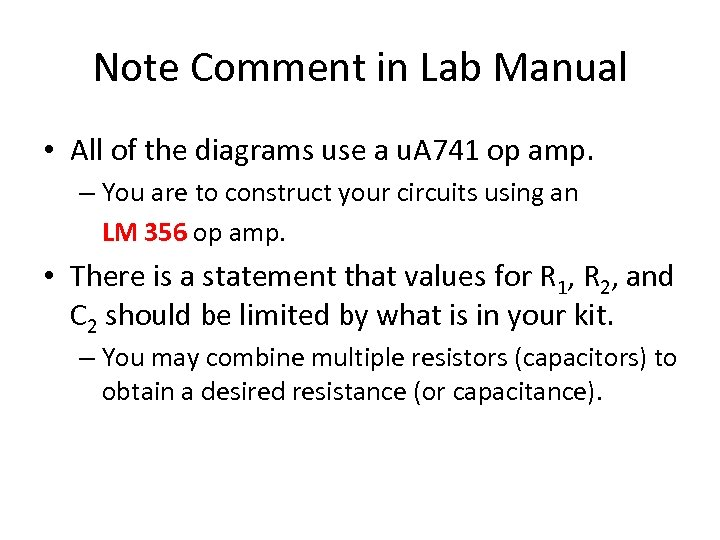Note Comment in Lab Manual • All of the diagrams use a u. A 741 op amp. – You are to construct your circuits using an LM 356 op amp. • There is a statement that values for R 1, R 2, and C 2 should be limited by what is in your kit. – You may combine multiple resistors (capacitors) to obtain a desired resistance (or capacitance).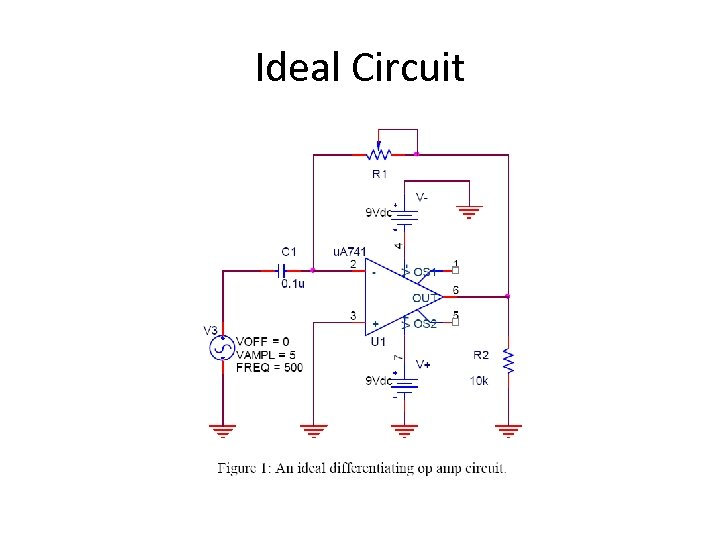Ideal CircuitCapacitors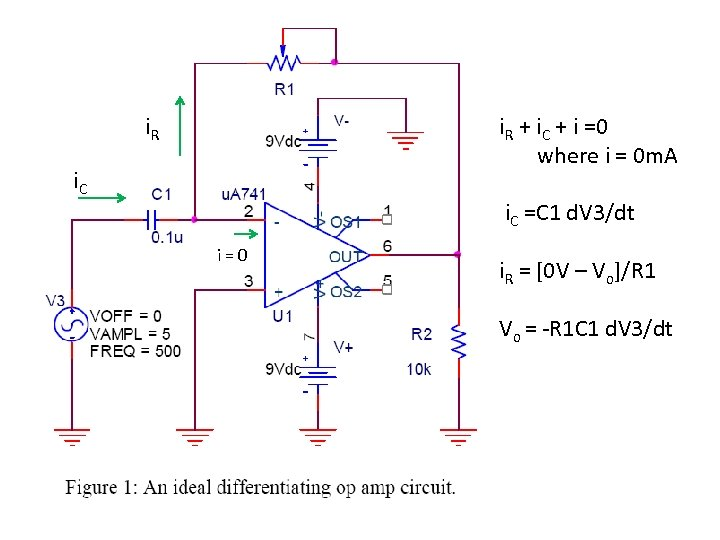i. R + i. C + i =0 where i = 0 m. A i. C =C 1 d. V 3/dt i=0 i. R = [0 V – Vo]/R 1 Vo = -R 1 C 1 d. V 3/dtPractical Circuit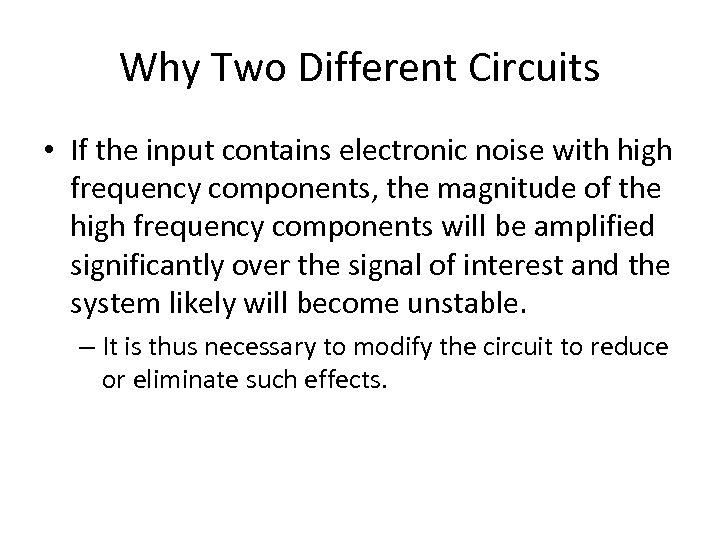Why Two Different Circuits • If the input contains electronic noise with high frequency components, the magnitude of the high frequency components will be amplified significantly over the signal of interest and the system likely will become unstable. – It is thus necessary to modify the circuit to reduce or eliminate such effects.Modifications to Ideal Circuit • Two modifications to the circuit – both of which results in the formation of frequency filters. – First, a series resistor is inserted before the negative input terminal of op amp. The effect of this resistor is to act as an attenuator for the high frequency components. – Second, a capacitor is placed in the feedback network. This capacitor provides more feed-back for the high frequency components than for the low frequency components and also acts to stabilize the circuit.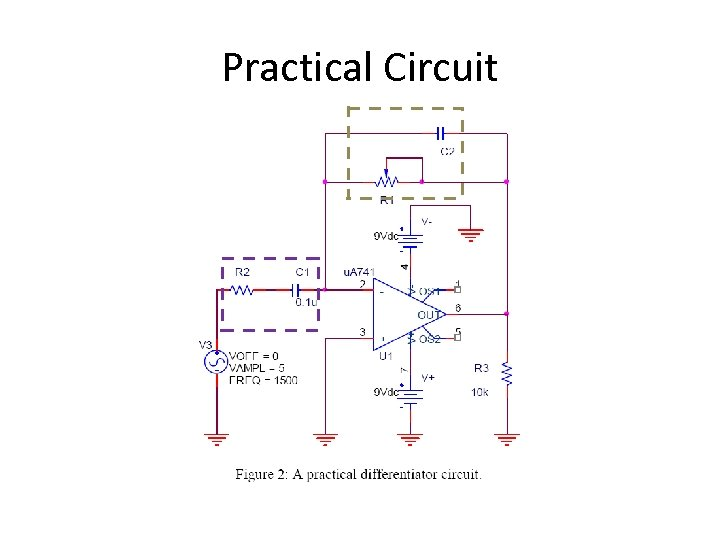Practical Circuit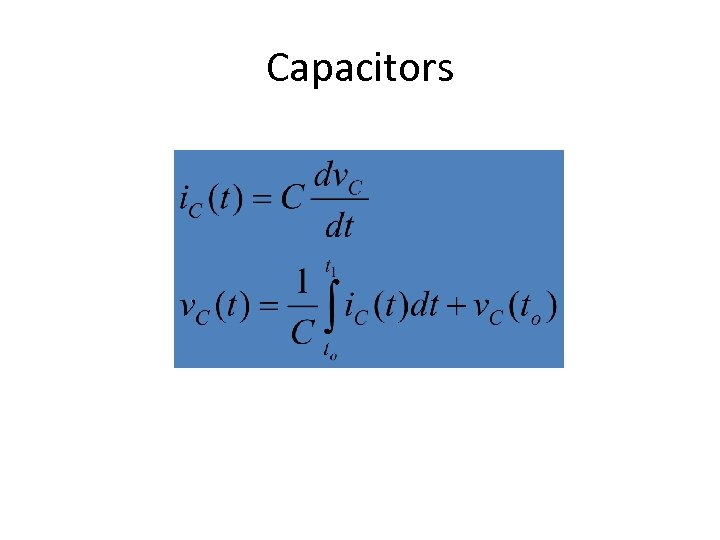Capacitors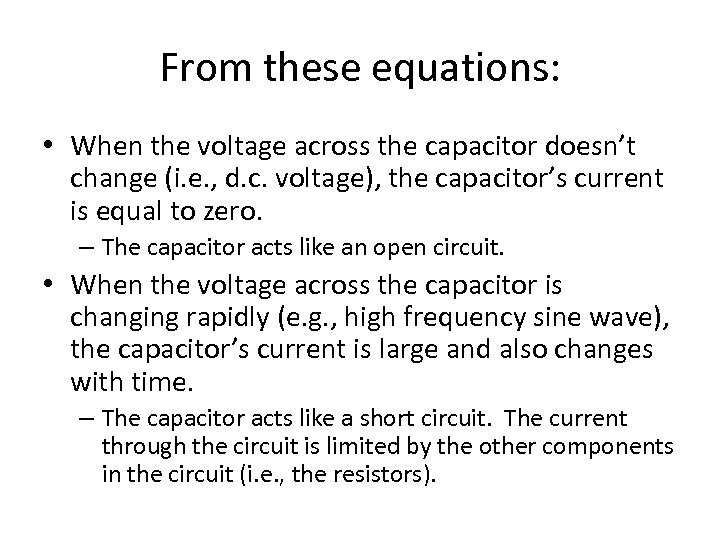From these equations: • When the voltage across the capacitor doesn’t change (i. e. , d. c. voltage), the capacitor’s current is equal to zero. – The capacitor acts like an open circuit. • When the voltage across the capacitor is changing rapidly (e. g. , high frequency sine wave), the capacitor’s current is large and also changes with time. – The capacitor acts like a short circuit. The current through the circuit is limited by the other components in the circuit (i. e. , the resistors).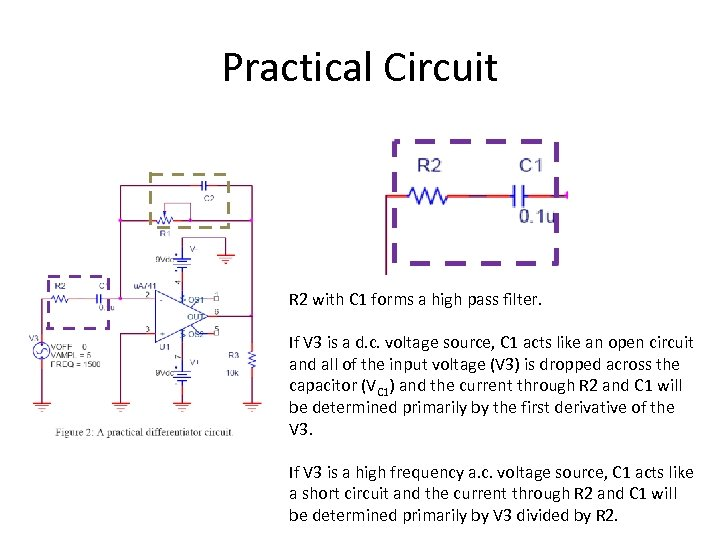Practical Circuit R 2 with C 1 forms a high pass filter. If V 3 is a d. c. voltage source, C 1 acts like an open circuit and all of the input voltage (V 3) is dropped across the capacitor (VC 1) and the current through R 2 and C 1 will be determined primarily by the first derivative of the V 3. If V 3 is a high frequency a. c. voltage source, C 1 acts like a short circuit and the current through R 2 and C 1 will be determined primarily by V 3 divided by R 2.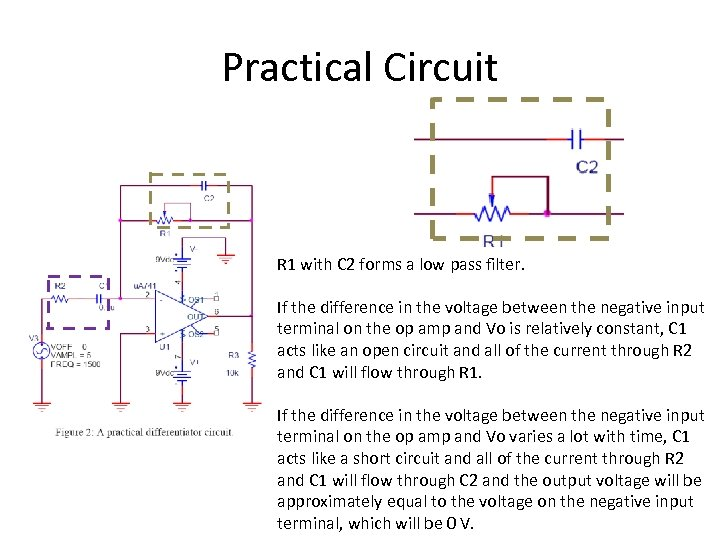Practical Circuit R 1 with C 2 forms a low pass filter. If the difference in the voltage between the negative input terminal on the op amp and Vo is relatively constant, C 1 acts like an open circuit and all of the current through R 2 and C 1 will flow through R 1. If the difference in the voltage between the negative input terminal on the op amp and Vo varies a lot with time, C 1 acts like a short circuit and all of the current through R 2 and C 1 will flow through C 2 and the output voltage will be approximately equal to the voltage on the negative input terminal, which will be 0 V.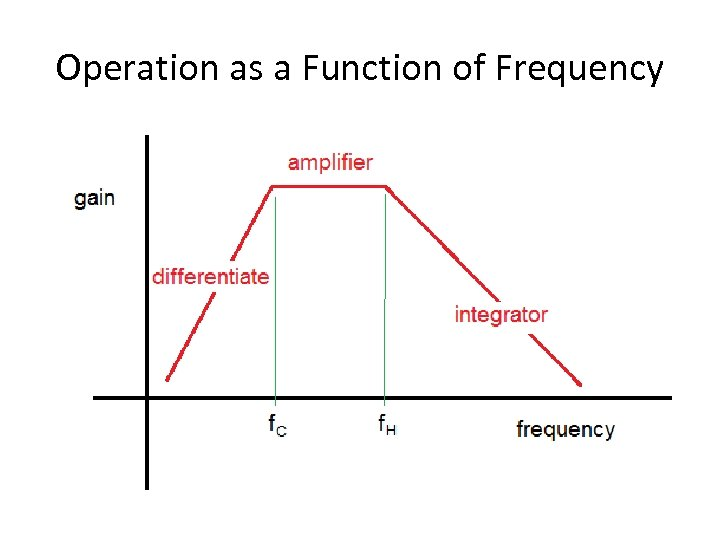Operation as a Function of Frequency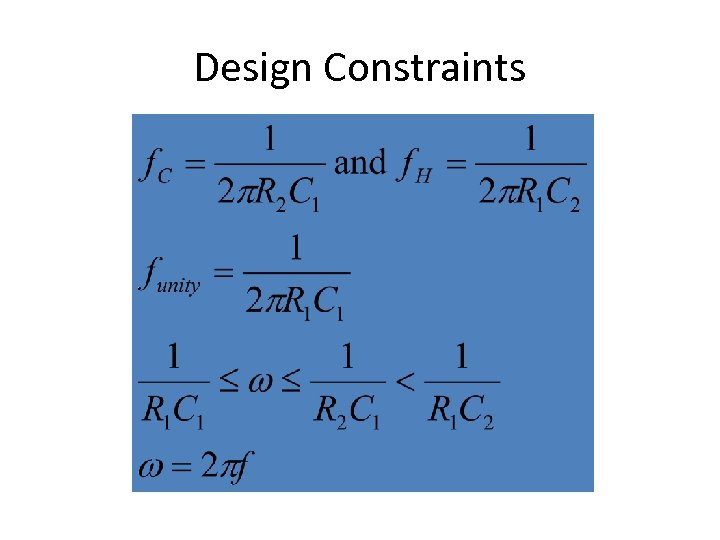Design ConstraintsDesign Constraints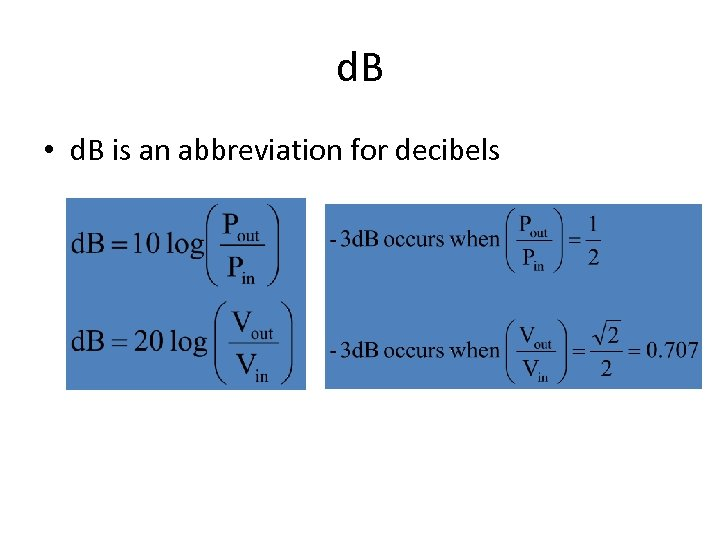d. B • d. B is an abbreviation for decibels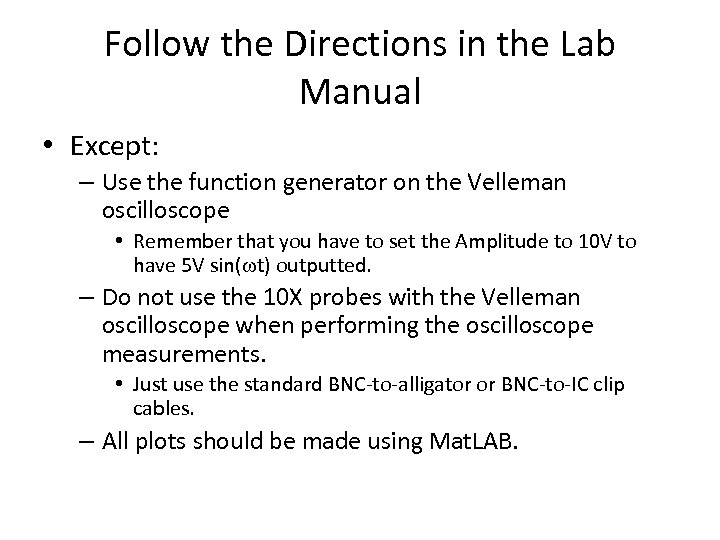Follow the Directions in the Lab Manual • Except: – Use the function generator on the Velleman oscilloscope • Remember that you have to set the Amplitude to 10 V to have 5 V sin(wt) outputted. – Do not use the 10 X probes with the Velleman oscilloscope when performing the oscilloscope measurements. • Just use the standard BNC-to-alligator or BNC-to-IC clip cables. – All plots should be made using Mat. LAB.PSpice Simulation: AC Sweep Differentiator Gain Integrator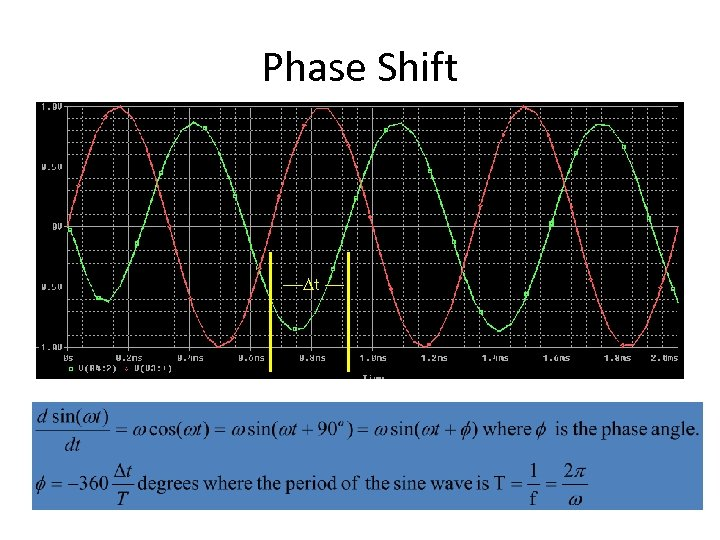Phase Shift --Dt --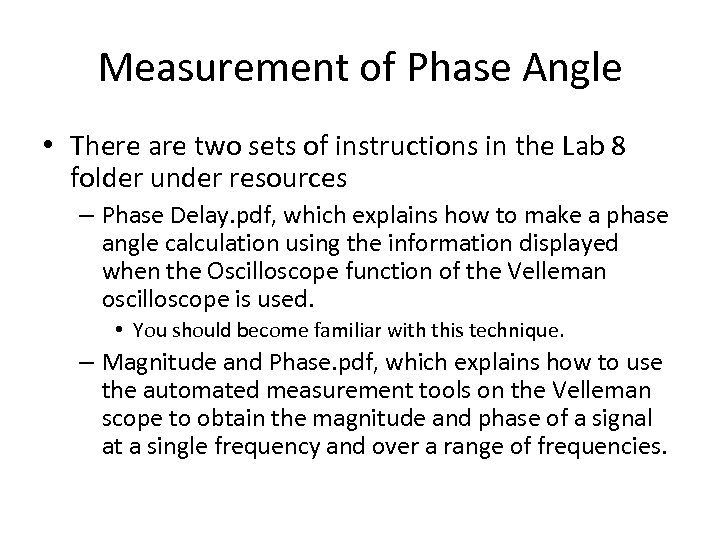Measurement of Phase Angle • There are two sets of instructions in the Lab 8 folder under resources – Phase Delay. pdf, which explains how to make a phase angle calculation using the information displayed when the Oscilloscope function of the Velleman oscilloscope is used. • You should become familiar with this technique. – Magnitude and Phase. pdf, which explains how to use the automated measurement tools on the Velleman scope to obtain the magnitude and phase of a signal at a single frequency and over a range of frequencies.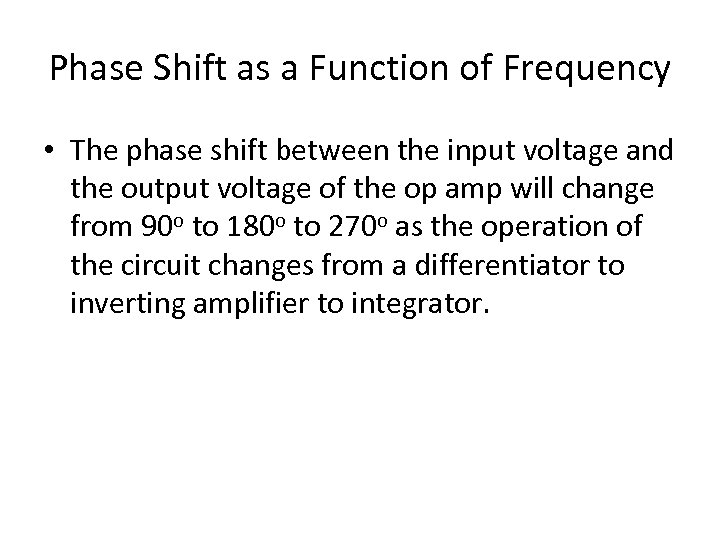Phase Shift as a Function of Frequency • The phase shift between the input voltage and the output voltage of the op amp will change from 90 o to 180 o to 270 o as the operation of the circuit changes from a differentiator to inverting amplifier to integrator.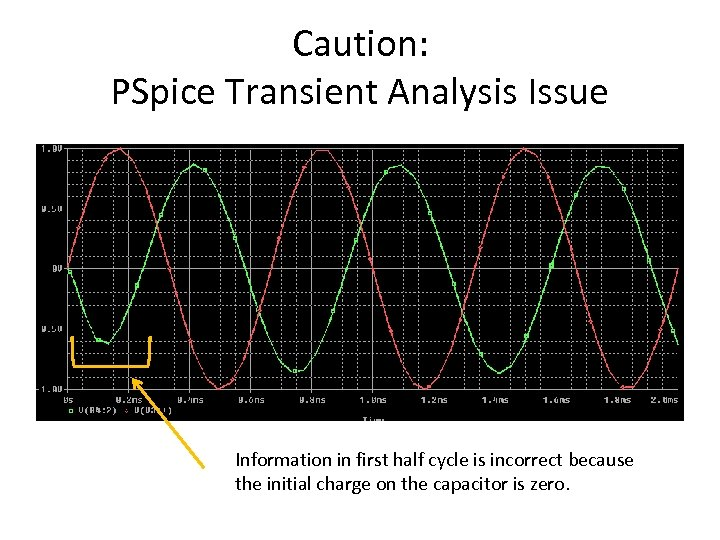Caution: PSpice Transient Analysis Issue Information in first half cycle is incorrect because the initial charge on the capacitor is zero.